This book is archived and will be removed July 6, 2022. Please use the updated version.

Chapter 8. Advanced Theories of Covalent Bonding

# 8.3 Multiple Bonds

### Learning Objectives

By the end of this section, you will be able to:

• Describe multiple covalent bonding in terms of atomic orbital overlap
• Relate the concept of resonance to π-bonding and electron delocalization

The hybrid orbital model appears to account well for the geometry of molecules involving single covalent bonds. Is it also capable of describing molecules containing double and triple bonds? We have already discussed that multiple bonds consist of σ and π bonds. Next we can consider how we visualize these components and how they relate to hybrid orbitals. The Lewis structure of ethene, C2H4, shows us that each carbon atom is surrounded by one other carbon atom and two hydrogen atoms.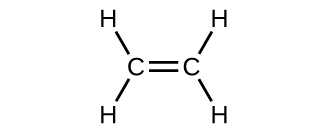The three bonding regions form a trigonal planar electron-pair geometry. Thus we expect the σ bonds from each carbon atom are formed using a set of sp2 hybrid orbitals that result from hybridization of two of the 2p orbitals and the 2s orbital (Figure 1). These orbitals form the C–H single bonds and the σ bond in the C=C double bond (Figure 2). The π bond in the C=C double bond results from the overlap of the third (remaining) 2p orbital on each carbon atom that is not involved in hybridization. This unhybridized p orbital (lobes shown in red and blue in Figure 2) is perpendicular to the plane of the sp2 hybrid orbitals. Thus the unhybridized 2p orbitals overlap in a side-by-side fashion, above and below the internuclear axis (Figure 2) and form a π bond.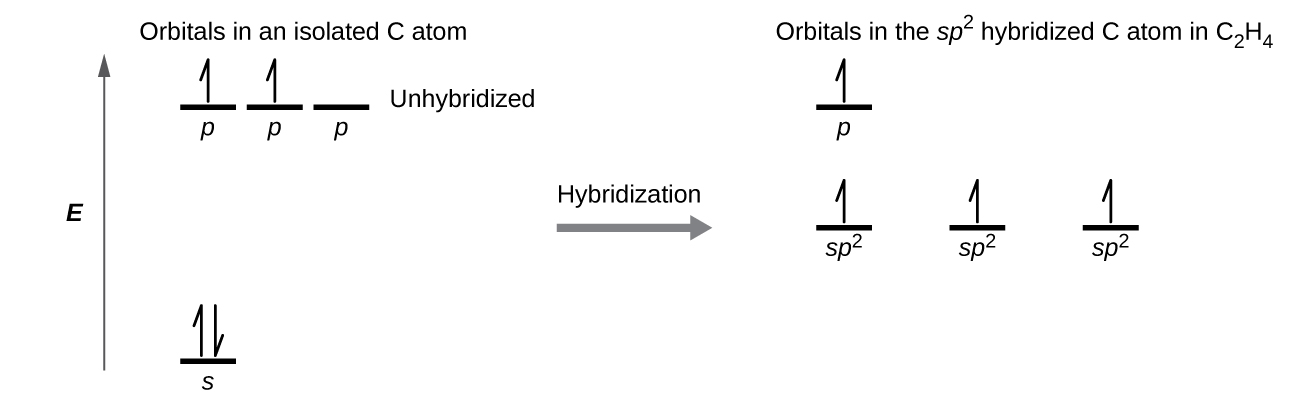Figure 1. In ethene, each carbon atom is sp2 hybridized, and the sp2 orbitals and the p orbital are singly occupied. The hybrid orbitals overlap to form σ bonds, while the p orbitals on each carbon atom overlap to form a π bond.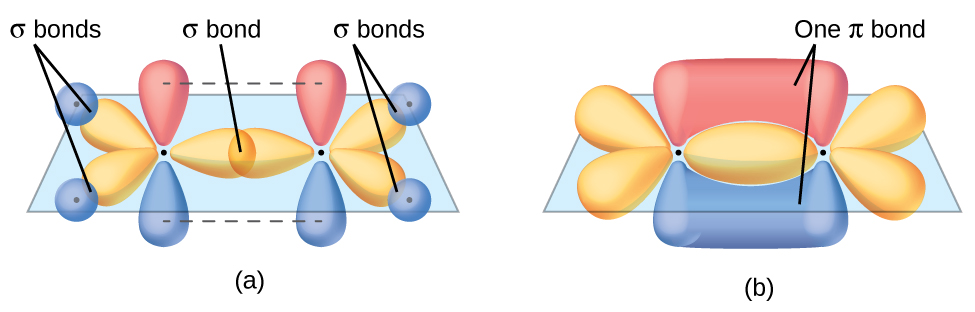Figure 2. In the ethene molecule, C2H4, there are (a) five σ bonds. One C–C σ bond results from overlap of sp2 hybrid orbitals on the carbon atom with one sp2 hybrid orbital on the other carbon atom. Four C–H bonds result from the overlap between the C atoms’ sp2 orbitals with s orbitals on the hydrogen atoms. (b) The π bond is formed by the side-by-side overlap of the two unhybridized p orbitals in the two carbon atoms. The two lobes of the π bond are above and below the plane of the σ system.

In an ethene molecule, the four hydrogen atoms and the two carbon atoms are all in the same plane. If the two planes of sp2 hybrid orbitals tilted relative to each other, the p orbitals would not be oriented to overlap efficiently to create the π bond. The planar configuration for the ethene molecule occurs because it is the most stable bonding arrangement. This is a significant difference between σ and π bonds; rotation around single (σ) bonds occurs easily because the end-to-end orbital overlap does not depend on the relative orientation of the orbitals on each atom in the bond. In other words, rotation around the internuclear axis does not change the extent to which the σ bonding orbitals overlap because the bonding electron density is symmetric about the axis. Rotation about the internuclear axis is much more difficult for multiple bonds; however, this would drastically alter the off-axis overlap of the π bonding orbitals, essentially breaking the π bond.

In molecules with sp hybrid orbitals, two unhybridized p orbitals remain on the atom (Figure 3). We find this situation in acetylene, H−C≡C−H, which is a linear molecule. The sp hybrid orbitals of the two carbon atoms overlap end to end to form a σ bond between the carbon atoms (Figure 4). The remaining sp orbitals form σ bonds with hydrogen atoms. The two unhybridized p orbitals per carbon are positioned such that they overlap side by side and, hence, form two π bonds. The two carbon atoms of acetylene are thus bound together by one σ bond and two π bonds, giving a triple bond.

Hybridization involves only σ bonds, lone pairs of electrons, and single unpaired electrons (radicals). Structures that account for these features describe the correct hybridization of the atoms. However, many structures also include resonance forms. Remember that resonance forms occur when various arrangements of π bonds are possible. Since the arrangement of π bonds involves only the unhybridized orbitals, resonance does not influence the assignment of hybridization.

For example, molecule benzene has two resonance forms (Figure 5). We can use either of these forms to determine that each of the carbon atoms is bonded to three other atoms with no lone pairs, so the correct hybridization is sp2. The electrons in the unhybridized p orbitals form π bonds. Neither resonance structure completely describes the electrons in the π bonds. They are not located in one position or the other, but in reality are delocalized throughout the ring. Valence bond theory does not easily address delocalization. Bonding in molecules with resonance forms is better described by molecular orbital theory. (See the next module.)

### Example 1

Assignment of Hybridization Involving Resonance
Some acid rain results from the reaction of sulfur dioxide with atmospheric water vapor, followed by the formation of sulfuric acid. Sulfur dioxide, SO2, is a major component of volcanic gases as well as a product of the combustion of sulfur-containing coal. What is the hybridization of the S atom in SO2?

Solution
The resonance structures of SO2 areThe sulfur atom is surrounded by two bonds and one lone pair of electrons in either resonance structure. Therefore, the electron-pair geometry is trigonal planar, and the hybridization of the sulfur atom is sp2.

Another acid in acid rain is nitric acid, HNO3, which is produced by the reaction of nitrogen dioxide, NO2, with atmospheric water vapor. What is the hybridization of the nitrogen atom in NO2? (Note: the lone electron on nitrogen occupies a hybridized orbital just as a lone pair would.)

sp2

# Key Concepts and Summary

Multiple bonds consist of a σ bond located along the axis between two atoms and one or two π bonds. The σ bonds are usually formed by the overlap of hybridized atomic orbitals, while the π bonds are formed by the side-by-side overlap of unhybridized orbitals. Resonance occurs when there are multiple unhybridized orbitals with the appropriate alignment to overlap, so the placement of π bonds can vary.

### Chemistry End of Chapter Exercises

1. The bond energy of a C–C single bond averages 347 kJ mol−1; that of a C≡C triple bond averages 839 kJ mol−1. Explain why the triple bond is not three times as strong as a single bond.
2. For the carbonate ion, CO32−, draw all of the resonance structures. Identify which orbitals overlap to create each bond.
3. A useful solvent that will dissolve salts as well as organic compounds is the compound acetonitrile, H3CCN. It is present in paint strippers.

(a) Write the Lewis structure for acetonitrile, and indicate the direction of the dipole moment in the molecule.

(b) Identify the hybrid orbitals used by the carbon atoms in the molecule to form σ bonds.

(c) Describe the atomic orbitals that form the π bonds in the molecule. Note that it is not necessary to hybridize the nitrogen atom.

4. For the molecule allene, H2C=C=CH2, give the hybridization of each carbon atom. Will the hydrogen atoms be in the same plane or perpendicular planes?
5. Identify the hybridization of the central atom in each of the following molecules and ions that contain multiple bonds:

(a) ClNO (N is the central atom)

(b) CS2

(c) Cl2CO (C is the central atom)

(d) Cl2SO (S is the central atom)

(e) SO2F2 (S is the central atom)

(f) XeO2F2 (Xe is the central atom)

(g) ClOF2+ (Cl is the central atom)

6. Describe the molecular geometry and hybridization of the N, P, or S atoms in each of the following compounds.

(a) H3PO4, phosphoric acid, used in cola soft drinks

(b) NH4NO3, ammonium nitrate, a fertilizer and explosive

(c) S2Cl2, disulfur dichloride, used in vulcanizing rubber

(d) K4[O3POPO3], potassium pyrophosphate, an ingredient in some toothpastes

7. For each of the following molecules, indicate the hybridization requested and whether or not the electrons will be delocalized:

(a) ozone (O3) central O hybridization

(b) carbon dioxide (CO2) central C hybridization

(c) nitrogen dioxide (NO2) central N hybridization

(d) phosphate ion (PO43−) central P hybridization

8. For each of the following structures, determine the hybridization requested and whether the electrons will be delocalized:

(a) Hybridization of each carbon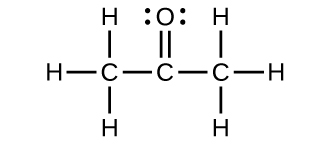(b) Hybridization of sulfur(c) All atoms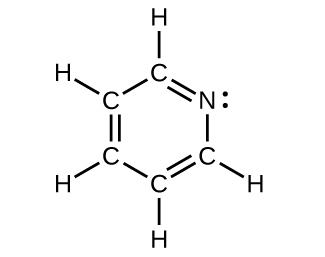9. Draw the orbital diagram for carbon in CO2 showing how many carbon atom electrons are in each orbital.

### Solutions

Answers to Chemistry End of Chapter Exercises

1. A triple bond consists of one σ bond and two π bonds. A σ bond is stronger than a π bond due to greater overlap.

3. (a)(b) The terminal carbon atom uses sp3 hybrid orbitals, while the central carbon atom is sp hybridized. (c) Each of the two π bonds is formed by overlap of a 2p orbital on carbon and a nitrogen 2p orbital.

5. (a) sp2; (b) sp; (c) sp2; (d) sp3; (e) sp3; (f) sp3d; (g) sp3

7. (a) sp2, delocalized; (b) sp, localized; (c) sp2, delocalized; (d) sp3, delocalized

9.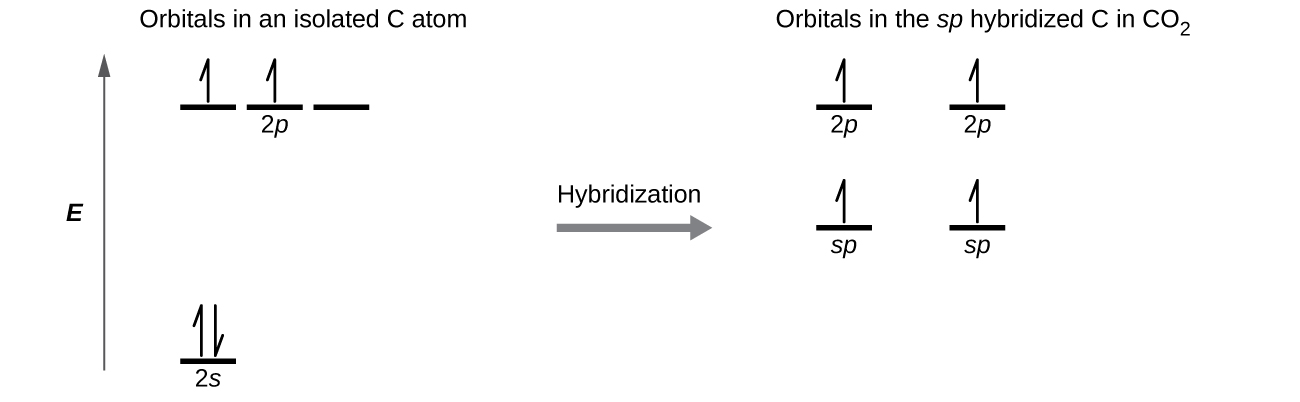Each of the four electrons is in a separate orbital and overlaps with an electron on an oxygen atom.